CBSE Class 10 Sample Paper for 2023 Boards - Maths Standard

Class 10
Solutions of Sample Papers for Class 10 Boards

## Due to heavy floods in a state, thousands were rendered homeless. 50 schools collectively decided to provide place and the canvas for 1500 tents and share the whole expenditure equally. The lower part of each tent is cylindrical with base radius 2.8 m and height 3.5 m and the upper part is conical with the same base radius, but of height 2.1 m. If the canvas used to make the tents costs ₹120 per m 2 , find the amount shared by each school to set up the tents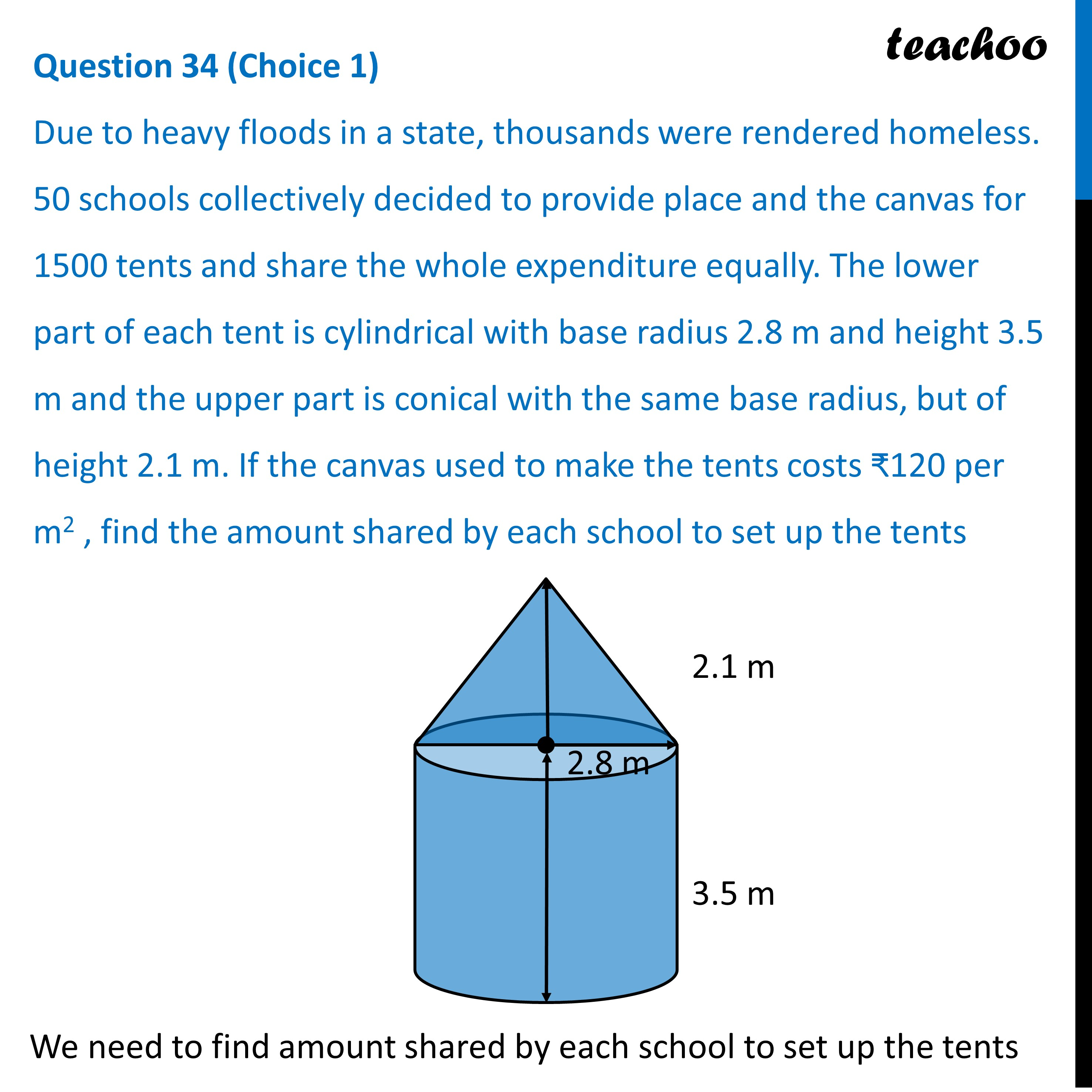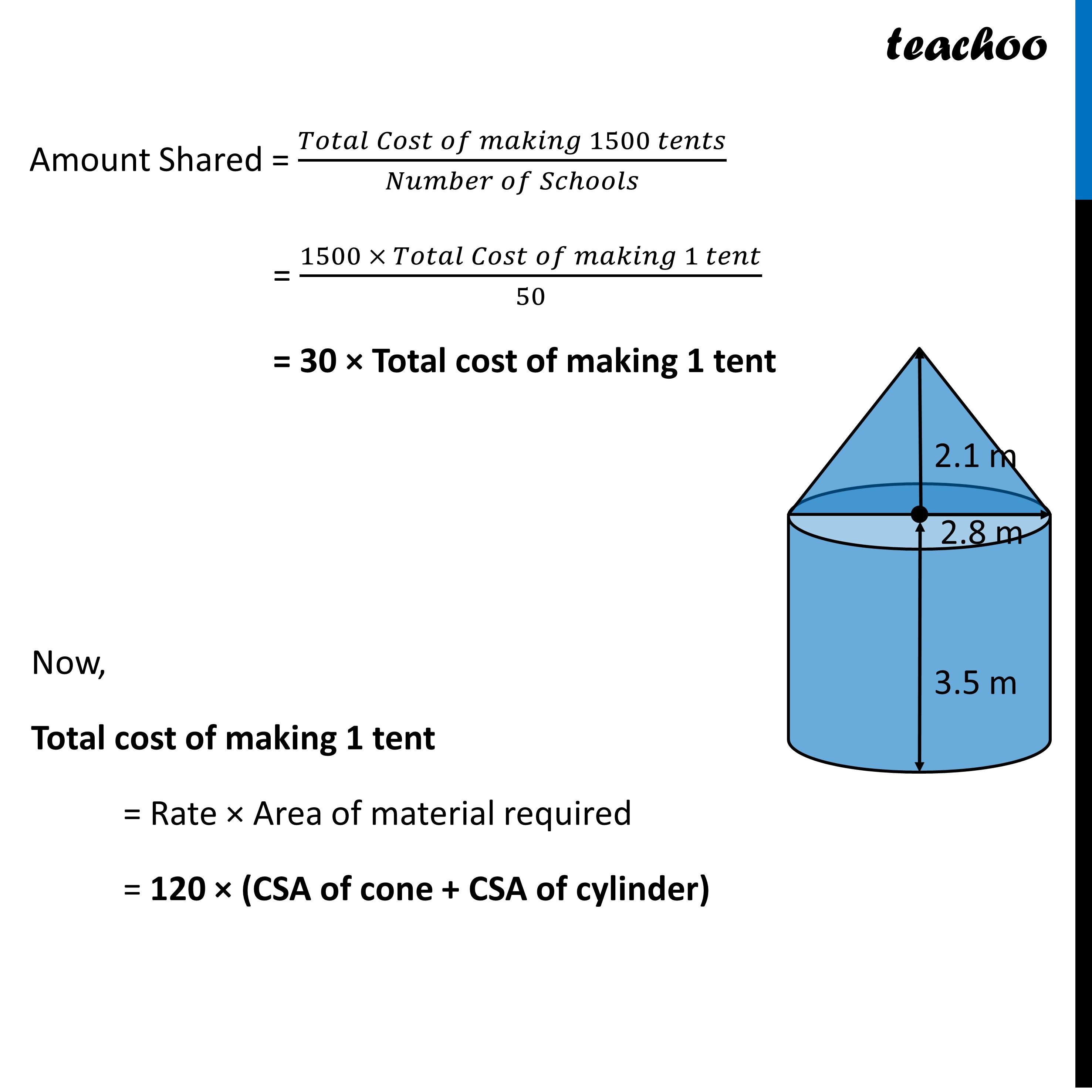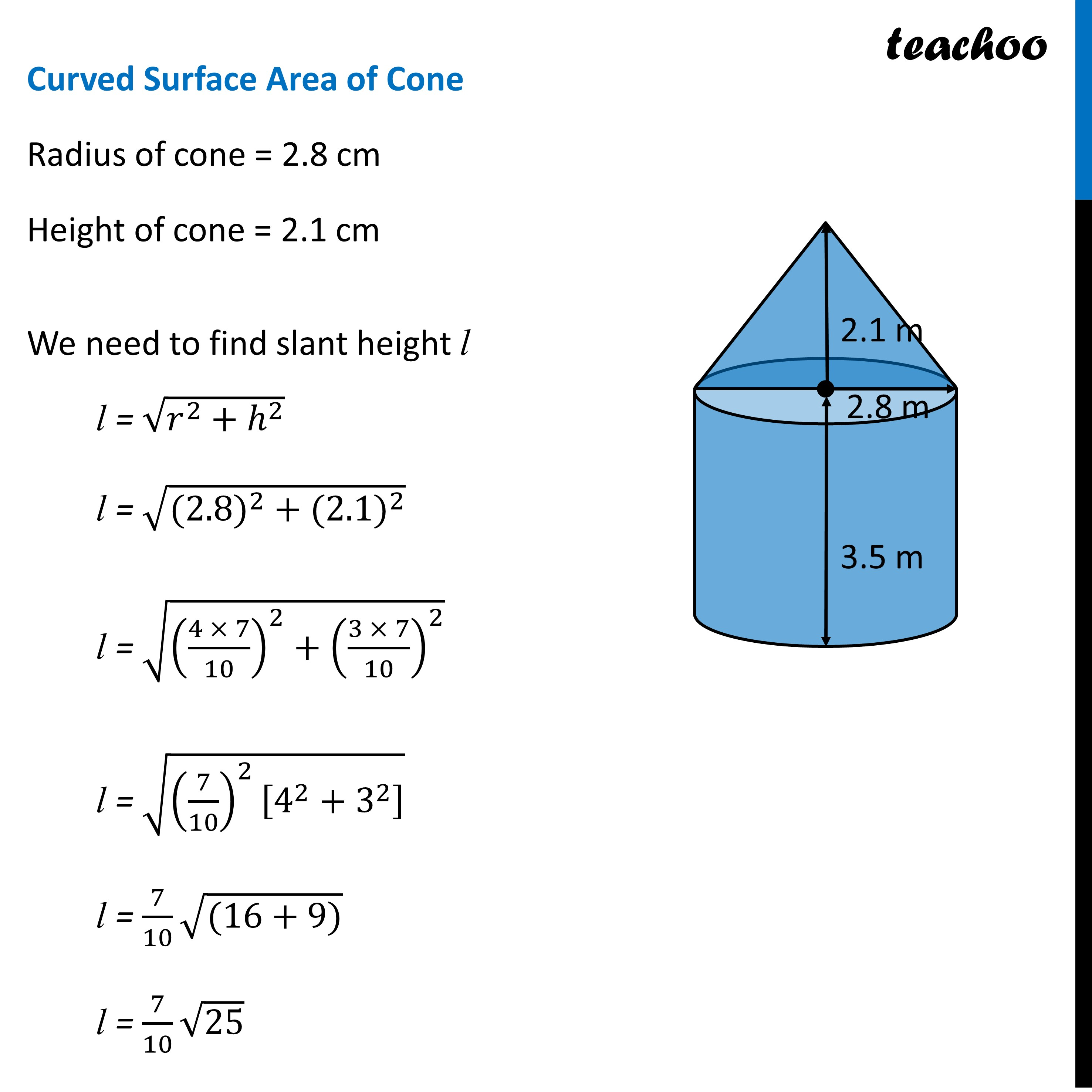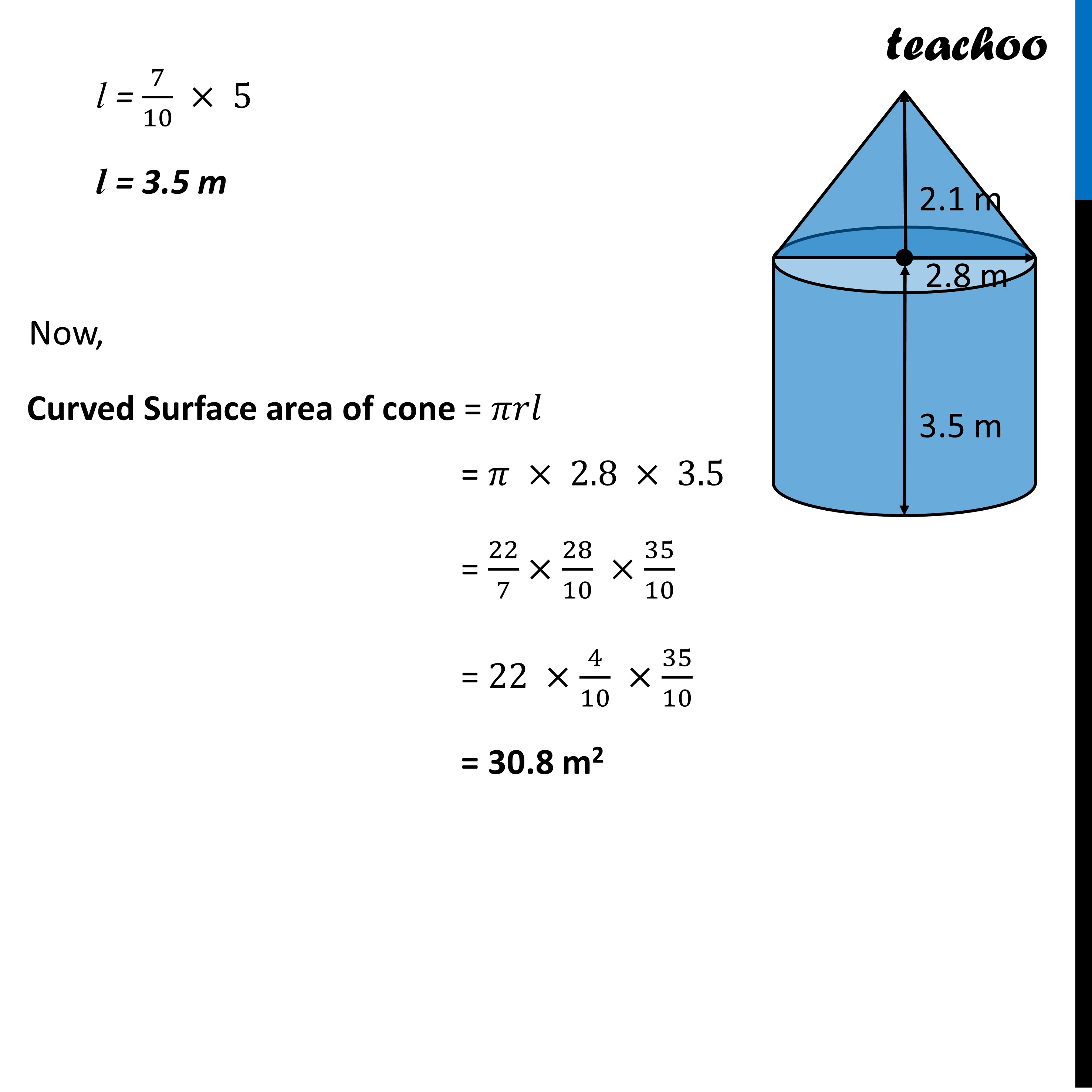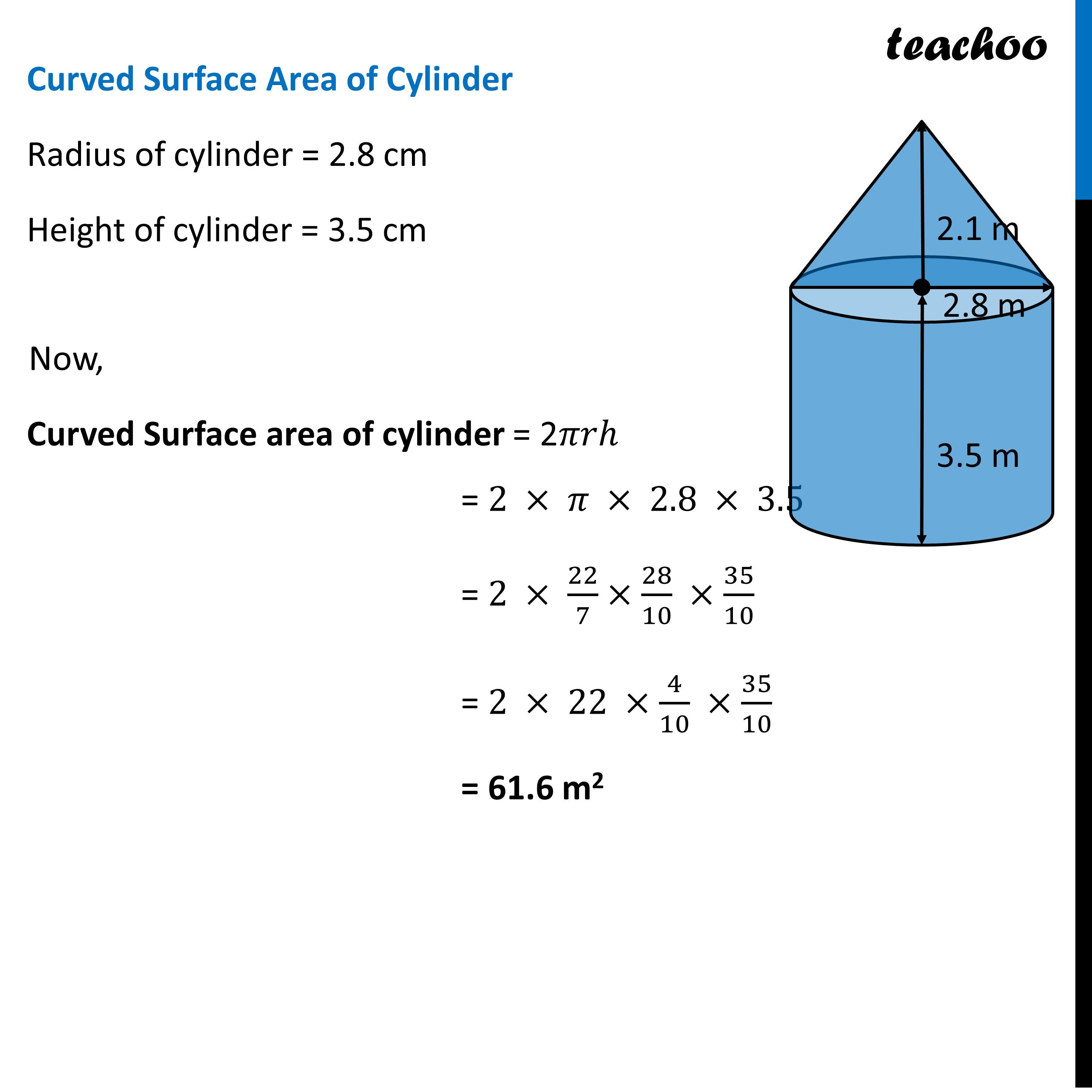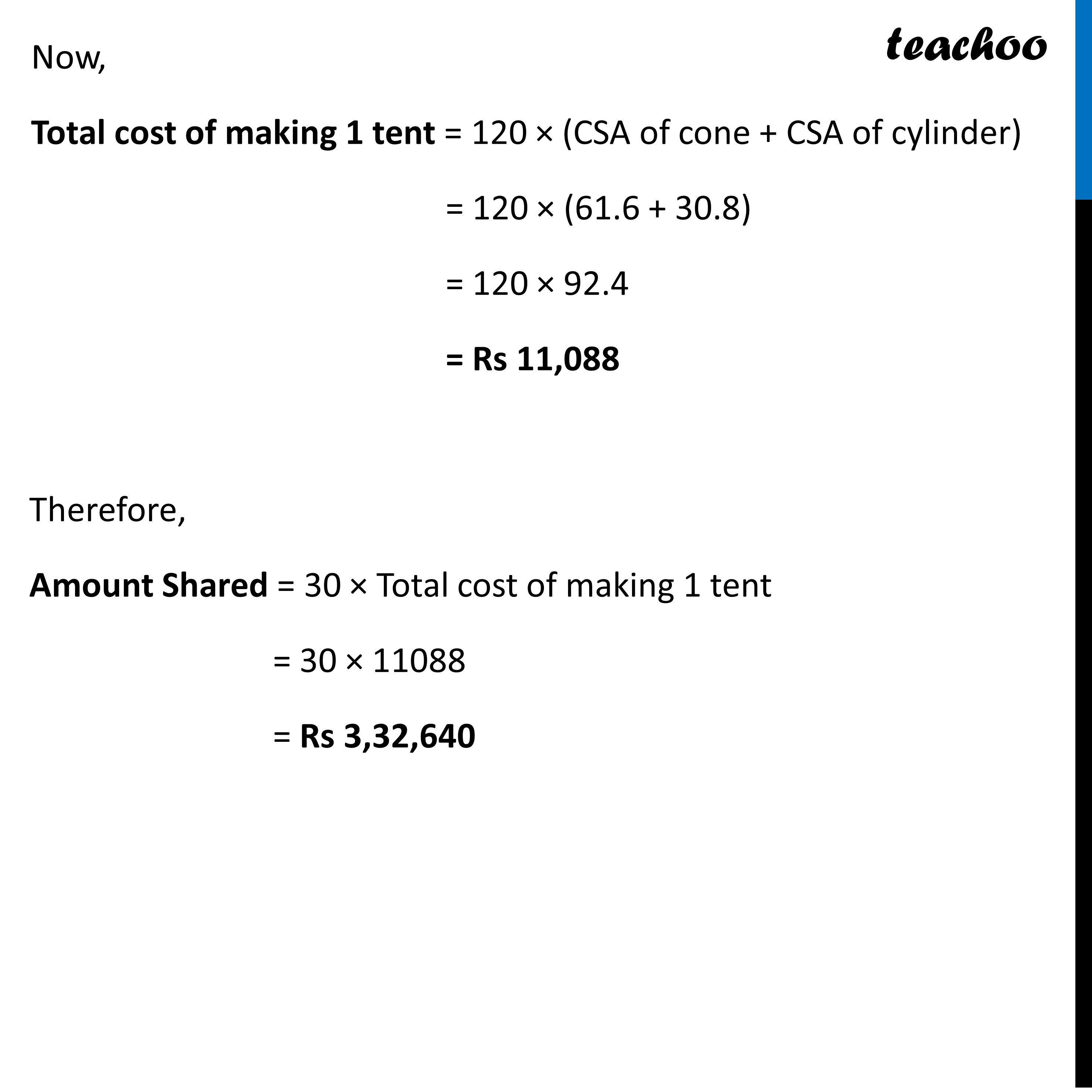Learn in your speed, with individual attention - Teachoo Maths 1-on-1 Class

### Transcript

Question 34 (Choice 1) Due to heavy floods in a state, thousands were rendered homeless. 50 schools collectively decided to provide place and the canvas for 1500 tents and share the whole expenditure equally. The lower part of each tent is cylindrical with base radius 2.8 m and height 3.5 m and the upper part is conical with the same base radius, but of height 2.1 m. If the canvas used to make the tents costs ₹120 per m2 , find the amount shared by each school to set up the tentsWe need to find amount shared by each school to set up the tents Amount Shared = (𝑇𝑜𝑡𝑎𝑙 𝐶𝑜𝑠𝑡 𝑜𝑓 𝑚𝑎𝑘𝑖𝑛𝑔 1500 𝑡𝑒𝑛𝑡𝑠)/(𝑁𝑢𝑚𝑏𝑒𝑟 𝑜𝑓 𝑆𝑐ℎ𝑜𝑜𝑙𝑠) = (1500 × 𝑇𝑜𝑡𝑎𝑙 𝐶𝑜𝑠𝑡 𝑜𝑓 𝑚𝑎𝑘𝑖𝑛𝑔 1 𝑡𝑒𝑛𝑡)/50 = 30 × Total cost of making 1 tent Now, Total cost of making 1 tent = Rate × Area of material required = 120 × (CSA of cone + CSA of cylinder)Curved Surface Area of Cone Radius of cone = 2.8 cm Height of cone = 2.1 cm We need to find slant height l l = √(𝑟^2+ℎ^2 ) l = √(〖(2.8)〗^2 + 〖(2.1)〗^2 ) l = √(((4 × 7)/10)^2+((3 × 7)/10)^2 ) l = √((7/10)^2 [4^2+3^2 ] ) l = 7/10 √((16+9)) l = 7/10 √25 l = 7/10 × 5 l = 3.5 m l = 7/10 × 5 l = 3.5 m Now, Curved Surface area of cone = 𝜋𝑟𝑙 = 𝜋 × 2.8 × 3.5 = 22/7×28/10 ×35/10 = 22 ×4/10 ×35/10 = 30.8 m2Curved Surface Area of Cylinder Radius of cylinder = 2.8 cm Height of cylinder = 3.5 cm Now, Curved Surface area of cylinder = 2𝜋𝑟ℎ = 2 × 𝜋 × 2.8 × 3.5 = 2 × 22/7×28/10 ×35/10 = 2 × 22 ×4/10 ×35/10 = 61.6 m2 Now, Total cost of making 1 tent = 120 × (CSA of cone + CSA of cylinder) = 120 × (61.6 + 30.8) = 120 × 92.4 = Rs 11,088 Therefore, Amount Shared = 30 × Total cost of making 1 tent = 30 × 11088 = Rs 3,32,640